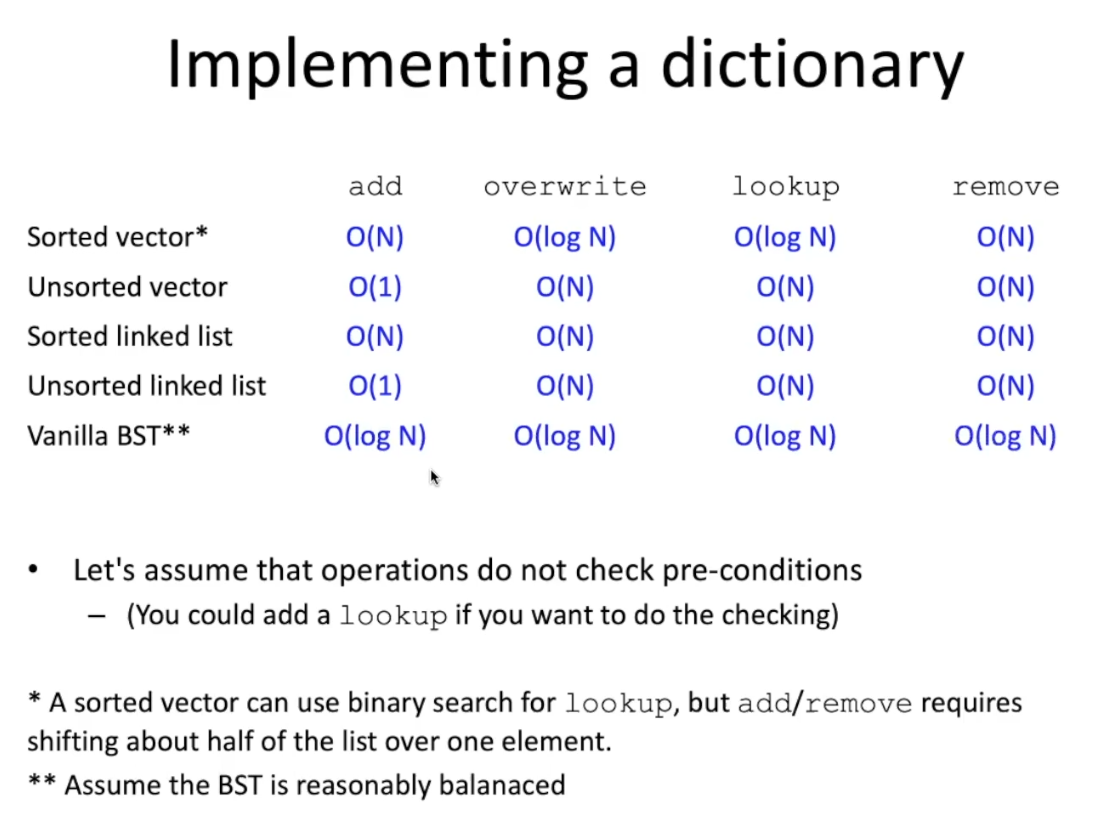Abstract Data Type

# Map §

A map is a generalized array that consists of key-value pairs. A dictionary is considered to be a Abstract Data Type.

### How to implement §### Built-in Implementation §

#### C++ §

Don’t use the square brackets (yet), use the other built-in functions that you can check here: https://en.cppreference.com/w/cpp/container/map insert something -> map::emplace get something -> map::at

##### map vs. unordered_map §
mapunordered_map
Orderingincreasing order (by default)no ordering
ImplementationSelf-Balancing Binary Search TreeHash Table
search time Average, Worst Case
Insertion time + RebalanceSame as search
Deletion time + RebalanceSame as search
map<string,int> m;
m["monkey"] = 4;
m["banana"] = 3;
m["harpsichord"] = 9;
cout << m["banana"] << "\n"; // 3

If the value of a key is requested but the map does not contain it, the key is automatically added to the map with a default value.

map<string,int> m;
cout << m["aybabtu"] << "\n"; // 0

The function count checks if a key exists in a map:

if (m.count("aybabtu")) {
// key exists
}

The following code prints all the keys and values in a map:

for (auto x : m) {
cout << x.first << " " << x.second << "\n";
}

Delete from a map

 std::map<char,int> mymap;
std::map<char,int>::iterator it;

// insert some values:
mymap['a']=10;
mymap['b']=20;
mymap['c']=30;
mymap['d']=40;
mymap['e']=50;
mymap['f']=60;

it=mymap.find('b');
mymap.erase (it);                   // erasing by iterator

mymap.erase ('c');                  // erasing by key

it=mymap.find ('e');
mymap.erase ( it, mymap.end() );    // erasing by range
###### Some other small insights §

Recently discovered that you don’t need to do this when counting.

// NOT NEEDED
if (m.count(x)) {
m[x]++;
} else {
m[x] = 1;
}

m[x]++;
a = {}
a['hi'] = True